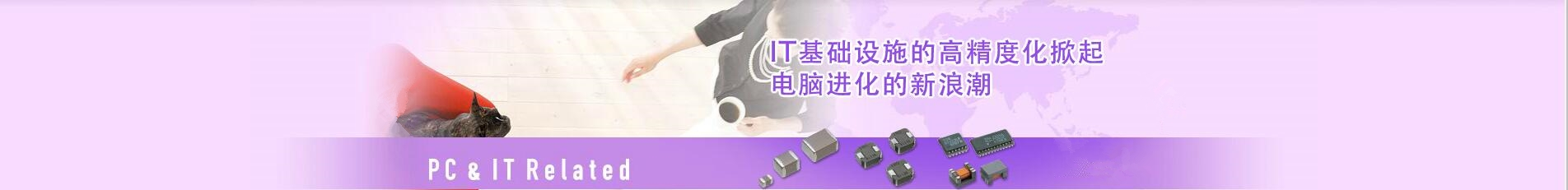## 日本TDK村田murata一级代理商-提供贴片电容器_电感器_蜂鸣器_滤波器_电阻磁珠_电源等产品代理服务

### 深圳市智成电子有限公司

Shenzhen Zhicheng Electronic Co., Ltd.

## 0755-23282269# 三星电容耐压值对照表三星贴片电容如何看电压

R=4V O=16V B=50V E=250V I=1000V Q=6.3V A=25V C=100V G=500V J=2000V P=10V L=35V D=200V H=630V K=3000V

1、系列编码：
CL=积层陶瓷电容
2、尺寸编码
03=0201（0603） 21=0805（2012） 42=1808（4520）
05=0402（1005） 31=1206（3216） 43=1812（4532）
10=0603（1608） 32=1210（3225） 55=2220（5750）
14=0504（1410） 01=0306（0816） 12=0508（1220）
3、介质
I类 II类
C=C0G S=S2H L=S2L
P=P2H T=T2H
R=R2H U=U2J
A=X5R F=Y5V
B=X7R X=X6S
4、容量

5、电容的误差：
B=±0.1pf F=±1pf±1% K=±10%
C=±0.25pf G=±2% M=±20%
D=±0.5pf J=±5% Z=+80/-20%
6、额定电压
R=4V O =16V B =50V E = 250V I = 1000V
Q=6.3V A =25V C=100V G = 500V J = 2000V
P =10V L =35V D =200V H = 630V K= 3000V
7、厚度：
3=0.30毫米 A=0.65毫米 M=1.15毫米 I=2.00毫米 Q=1.25毫米
5=0.50毫米 C=0.85毫米 F=1.25毫米 J=2.50毫米 V=2.50毫米
8=0.80毫米 D=1.00毫米 H=1.60毫米 L=3.20毫米
8、内电极
A=常规产品 钯/银/镍屏蔽/锡 100%
N=常规产品 镍/铜/镍屏蔽/锡 100%
G=常规产品 铜/铜/镍屏蔽/锡 100%
L=低侧面产品 镍/铜/镍屏蔽/锡 100%
9、产品编码
A =阵列（2-元素） L =LICC
B =阵列（4-元素） N =常规
P =自动 C=高频
10、特殊编码
11、包装编码
B=散装 O=纸版箱料带，10英寸料盘 E=压花纸版箱，7英寸料盘
P=散装箱 D=纸版箱料带，13英寸料盘（10000ea） F=压花纸版箱，13英寸料盘
C=纸版箱料带，7英寸料盘 L=纸版箱料带，13英寸料盘（15，000ea） S=压花纸版箱，10英寸料盘

0755-23282269